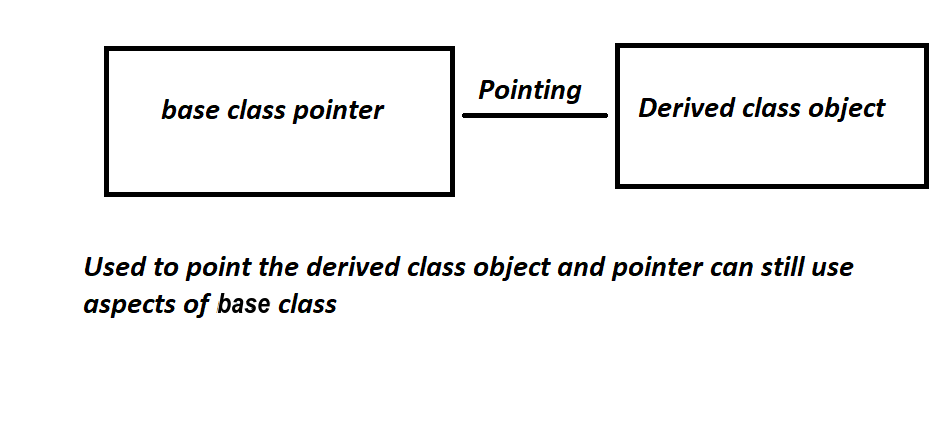GeeksforGeeks App
Open AppBrowser
Continue

# Base Class Pointer Pointing to Derived Class Object in C++

Prerequisite: Pointers in C++

A pointer is a data type that stores the address of other data types. Pointers can be used for base objects as well as objects of derived classes. A pointer to the object of the derived class and a pointer to the object of the base class are type-compatible (may be used in different ways).

The pointer of Base Class pointing different objects of the derived classBase class pointer to Derived class object

## Approach:

• A derived class is a class that takes some properties from its base class.
• It is true that a pointer of one class can point to another class, but classes must be a base and derived class, then it is possible.
• To access the variable of the base class, a base class pointer will be used.
• So, a pointer is a type of base class, and it can access all, public function and variables of the base class since the pointer is of the base class, this is known as a binding pointer.
• In this pointer base class is owned by the base class but points to the derived class object.
• The same works with derived class pointer, values are changed.

Example:

## C++

 `// C++ program to Demonstrate the``// implementation of the base class``// pointer pointing to derived class``#include ``using` `namespace` `std;` `// Base Class``class` `BaseClass {``public``:``    ``int` `var_base;` `    ``// Function to display the base``    ``// class members``    ``void` `display()``    ``{``        ``cout << ``"Displaying Base class"``             ``<< ``" variable var_base: "` `<< var_base << endl;``    ``}``};` `// Class derived from the Base Class``class` `DerivedClass : ``public` `BaseClass {``public``:``    ``int` `var_derived;` `    ``// Function to display the base``    ``// and derived class members``    ``void` `display()``    ``{``        ``cout << ``"Displaying Base class"``             ``<< ``"variable var_base: "` `<< var_base << endl;``        ``cout << ``"Displaying Derived "``             ``<< ``" class variable var_derived: "``             ``<< var_derived << endl;``    ``}``};` `// Driver Code``int` `main()``{``    ``// Pointer to base class``    ``BaseClass* base_class_pointer;``    ``BaseClass obj_base;``    ``DerivedClass obj_derived;` `    ``// Pointing to derived class``    ``base_class_pointer = &obj_derived;` `    ``base_class_pointer->var_base = 34;` `    ``// If you uncomment this line of code this will cause``    ``// the following error As base-class pointer cannot``    ``// access the derived class variable. ``    ``// base_class_pointer->var_derived = 98;``    ``// output: error: ‘class BaseClass’ has no member named``    ``// ‘var_derived’` `    ``// Calling base class member function``    ``base_class_pointer->display();` `    ``base_class_pointer->var_base = 3400;``    ``base_class_pointer->display();` `    ``DerivedClass* derived_class_pointer;``    ``derived_class_pointer = &obj_derived;``    ``derived_class_pointer->var_base = 9448;``    ``derived_class_pointer->var_derived = 98;``    ``derived_class_pointer->display();` `    ``return` `0;``}`

Output

```Displaying Base class variable var_base: 34
Displaying Base class variable var_base: 3400
Displaying Base classvariable var_base: 9448
Displaying Derived  class variable var_derived: 98```

Time Complexity: O(1).

Space Complexity: O(1).

## How is Upcasting related to Base class pointer pointing to derived class object?

A derived-class reference or pointer is Upcast to a base class. To put it another way, upcasting enables us to handle derived types as if they were their base types.

Without an explicit typecast, public inheritance is always permitted. This is a result of the base and derived classes having an is-a relationship.

Although C++ permits a base pointer to point to any object derived from that base, The pointer cannot be directly used to access all the members of the derived class we may have to use another pointer declared as a pointer to the derived type.

## Conclusion:

• A pointer to a derived class is a pointer of a base class pointing to a derived class, but it will hold its aspect.
• This pointer of the base class will be able to temper functions and variables of its own class and can still point to the derived class object.

My Personal Notes arrow_drop_up